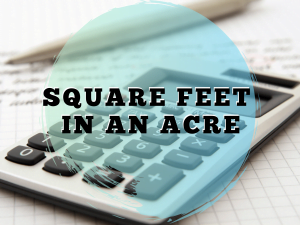Monday, October 2, 2023

# Acre to Sq ft | How much is an acre in square feet | Sq ft to Acre

In this article, we will discuss the conversion of an acre to sq ft and sq ft to the acre.

### 1. Introduction

An acre is a traditional measurement unit of land area, depending on basis of imperial or US customary measurement system. Acre is utilized for land measurement, used by farmers, and in real estate for land purchase and sale of residential and commercial plots.

The acre is explained as an area of land of 1 chain by one furlong as 66 feet by 660 feet, which is equal to 10 square Chain, 1/640 of a square mile or 43560 square feet, and exact 4047 square meters or 4840 square yards which is equal as about 40% of a hectare in the metric system of land measurement.

### 2. Acre to sq ft

An area of Land of 1 chain by 1 furlong, such as 1 acre = 1 chain × 1 furlong, which is equal to 45 feet by 968 feet or 43560 square feet, so, therefore, to convert acre to sq ft conversion you need to know the value of 1 acre = 43560 sq ft.

#### a. Acre to sqft conversion

Here, 1 acre = 1 chain × 1 furlong,

As we know 1 furlong equals 10 chains, so we have

1 acre = 1 chain × 10 chains,

As we know that one chain is equal to 22 yards so we have

1 acre = 22 yards × 22 yards,

1 acre = 4840 sq. yards,

As we know that one square yard is equal to 9 square feet so we have,

1 acre = 9× 4840 sq. ft,

Hence, 1 acre = 43560 sq. ft.#### b. Sq ft to Acre Conversion

Suppose an area of 100 feet by 200 feet of land is equal to 20000 sq ft, as

1 acre = 43560 sq ft,

= 20000/43560

= 0.459 acres.

### 3. Acre to Sq ft Table

 Acres Sq.ft 0.8 34848 1 43560 1.1 47916 2 87120 2.1 91476 3 130680 3.1 135036 4 174240 4.1 178596 5 217800

 Read Also: Units in Civil EngineeringHe is a founder and lead author of Dream Civil International and his civil engineering research articles has been taken as source by world's top news and educational sites like USA Today, Time, The richest, Wikipedia, etc.
Latest Articles

Related Articles